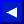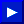When the NEXT is reached, the counter value is incremented by 1 or by an optional STEP value. It is then tested against an end-value to see if it's time to stop the loop. A loop will be stopped when a NEXT is found which has its counter value greater than the end-value.

EXAMPLES of NEXT Statement:
```   10 FOR J=1 TO 5: FOR K=10 TO 20: FOR N=5 TO -5 STEP - 1

20 NEXT N,K,J            (Stopping Nested Loops)

10 FOR L=1 TO 100
20 FOR M=1 TO 10
30 NEXT M
400 NEXT L               (Note how the loops do NOT cross each other)

10 FOR A=1 TO 10
20 FOR B=1 TO 20
30 NEXT
40 NEXT                  (Notice that no variable names are needed)
```

### NOT

TYPE: Logical Operator
FORMAT: NOT <expression>

Action: The NOT logical operator "complements" the value of each bit in its single operand, producing an integer "twos-complement" result. In other words, the NOT is really saying, "if it isn't. When working with a floating-point number, the operands are converted to integers and any fractions are lost. The NOT operator can also be used in a comparison to reverse the true/false value which was the result of a relationship test and therefore it will reverse the meaning of the comparison. In the first example below, if the "twos-complement" of "AA" is equal to "BB" and if "BB" is NOT equal to "CC" then the expression is true.This page has been created by Sami Rautiainen. Read the small print. Last updated November 14, 1998.